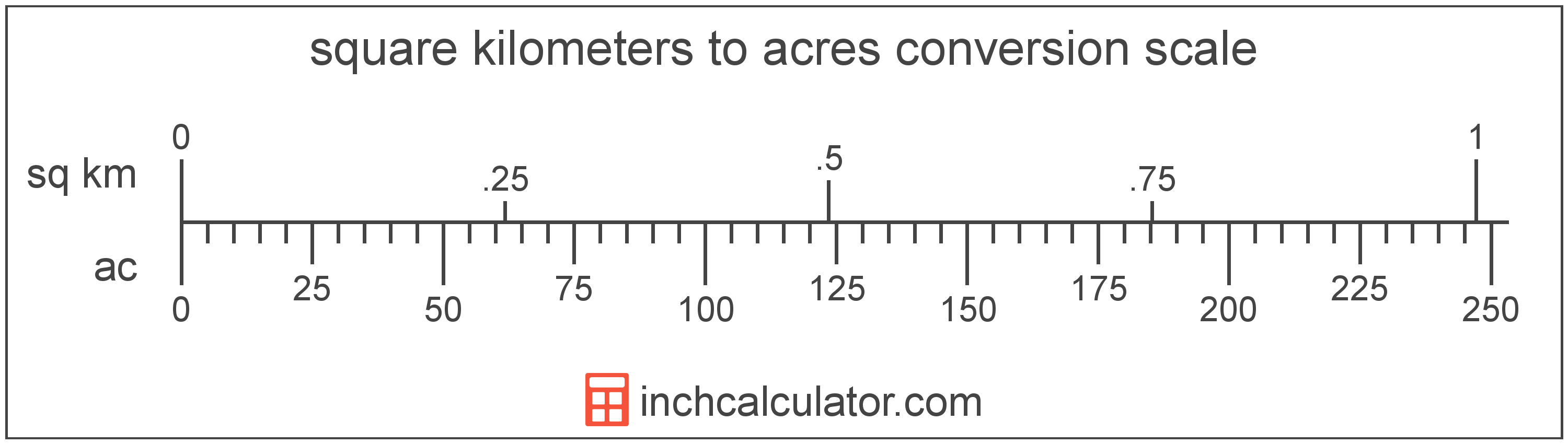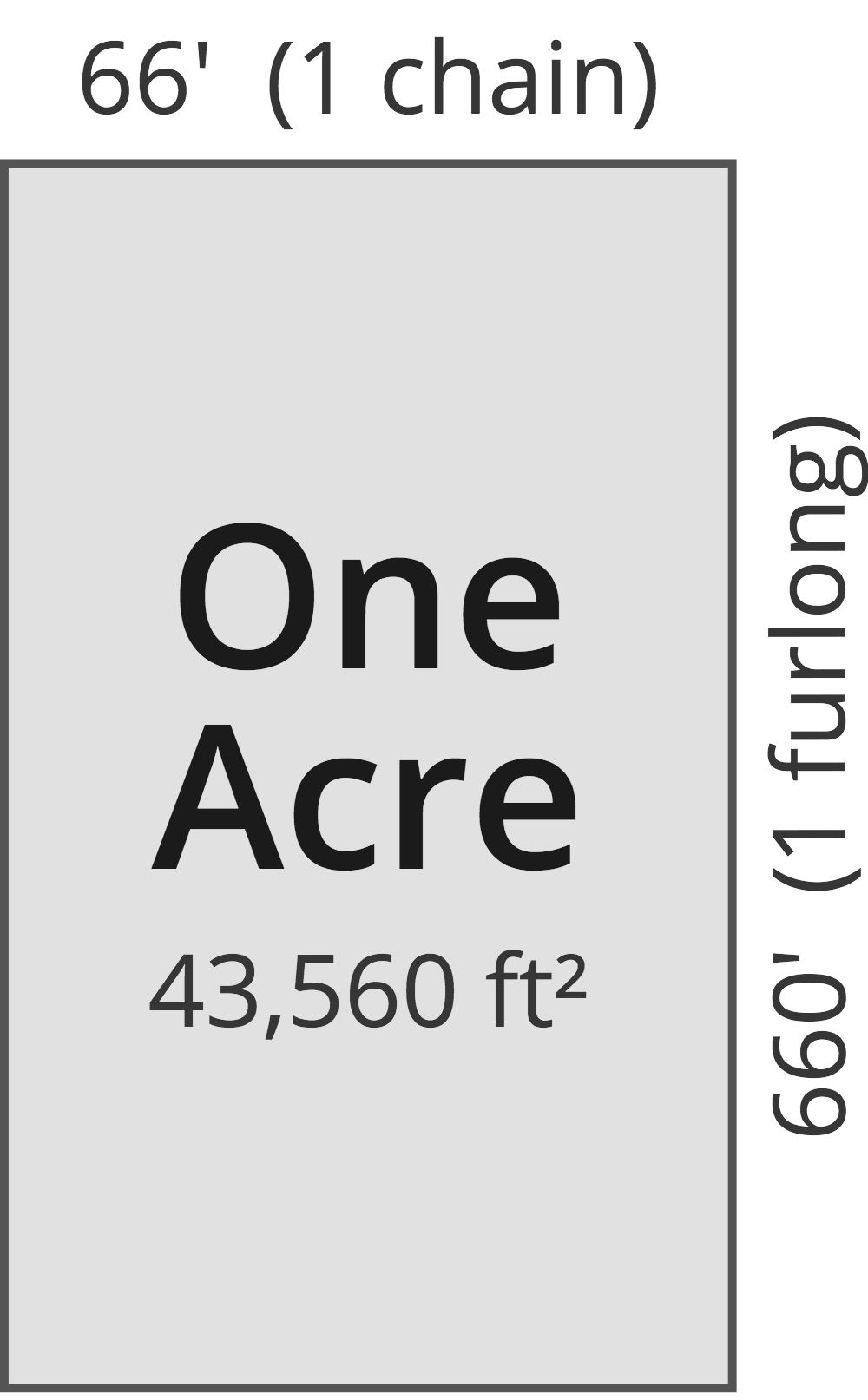# Acres to Square Kilometers Converter

Enter the area in acres below to get the value converted to square kilometers.

(find acres)
Results in Square Kilometers:1 ac = 0.004047 sq km

Do you want to convert square kilometers to acres?

## How to Convert Acres to Square Kilometers

To convert a measurement in acres to a measurement in square kilometers, multiply the area by the following conversion ratio: 0.004047 square kilometers/acre.

Since one acre is equal to 0.004047 square kilometers, you can use this simple formula to convert:

square kilometers = acres × 0.004047

The area in square kilometers is equal to the area in acres multiplied by 0.004047.

For example, here's how to convert 500 acres to square kilometers using the formula above.
square kilometers = (500 ac × 0.004047) = 2.023428 sq km## What is an Acre?

One acre is defined as the area equal to a space that is one chain (66 ft) by one furlong (660 ft), or 10 square chains. That's equal to 43,560 square feet or 1/640 of a square mile for those unfamiliar with those units of measure.The acre is a US customary and imperial unit of area. Acres can be abbreviated as ac; for example, 1 acre can be written as 1 ac.

You can use an acreage calculator to measure the area of a plot of land in acres by locating the boundaries on a map.

## What is a Square Kilometer?

One square kilometer is equivalent to the area of a square with sides that are each 1 kilometer in length. One square kilometer is equal to 0.386102 square miles or 1,000,000 square meters.

The square kilometer, or square kilometre, is a multiple of the square meter, which is the SI derived unit for area. In the metric system, "kilo" is the prefix for thousands, or 103. A square kilometer is sometimes also referred to as a square km. Square kilometers can be abbreviated as sq km, and are also sometimes abbreviated as km². For example, 1 square kilometer can be written as 1 sq km or 1 km².

## Acre to Square Kilometer Conversion Table

Table showing various acre measurements converted to square kilometers.
Acres Square Kilometers
1 ac 0.004047 sq km
2 ac 0.008094 sq km
3 ac 0.012141 sq km
4 ac 0.016187 sq km
5 ac 0.020234 sq km
6 ac 0.024281 sq km
7 ac 0.028328 sq km
8 ac 0.032375 sq km
9 ac 0.036422 sq km
10 ac 0.040469 sq km
20 ac 0.080937 sq km
30 ac 0.121406 sq km
40 ac 0.161874 sq km
50 ac 0.202343 sq km
60 ac 0.242811 sq km
70 ac 0.28328 sq km
80 ac 0.323749 sq km
90 ac 0.364217 sq km
100 ac 0.404686 sq km
200 ac 0.809371 sq km
300 ac 1.2141 sq km
400 ac 1.6187 sq km
500 ac 2.0234 sq km
600 ac 2.4281 sq km
700 ac 2.8328 sq km
800 ac 3.2375 sq km
900 ac 3.6422 sq km
1,000 ac 4.0469 sq km

## References

1. National Institute of Standards and Technology, Specifications, Tolerances, and Other Technical Requirements for Weighing and Measuring Devices, Handbook 44 - 2019 Edition, https://nvlpubs.nist.gov/nistpubs/hb/2019/NIST.HB.44-2019.pdf